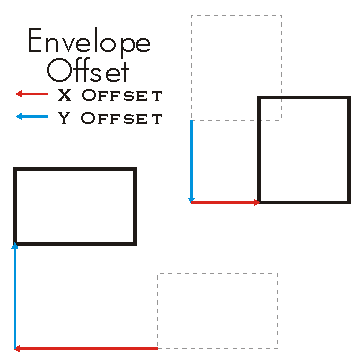ArcObjects Library Reference (Geometry)

# IEnvelope.Offset Method

Moves the sides x units horizontally and y units vertically.

```[Visual Basic .NET]
Public Sub Offset ( _
ByVal X As Double, _
ByVal Y As Double _
)```
```[C#]
public void Offset (
double X,
double Y
);```
```[C++]
HRESULT Offset(
double X,
double Y
);```
```[C++]
Parameters
X
X is a parameter of type double
Y
Y is a parameter of type double
```

#### Product Availability

Available with ArcGIS Engine, ArcGIS Desktop, and ArcGIS Server.

#### Description

Offset moves the position of the Envelope.  A positive X value will shift the Envelope to the right and a negative value to the left. A positive Y value will shift the Envelope up and a negative value down.  The Width and Height of the Envelope remain unchanged.  Only the XMin, YMin, XMax, and YMax are changed.

#### Remarks

The new position of the Envelope is as follows:

new XMin= old XMin + X
new YMin = old YMin + Y
new XMax = old XMax + X
new YMax = old YMax + Y[C#]

private void SetOffset()
{
IEnvelope envelope = new EnvelopeClass();
envelope.PutCoords(100, 100, 200, 200);

String report1 = "Envelope before setting offset: \n" +
"LowerLeft  X = " + envelope.LowerLeft.X + "\n" +
"LowerLeft  Y = " + envelope.LowerLeft.Y + "\n\n" +
"LowerRight X =  " + envelope.LowerRight.X + "\n" +
"LowerRight Y =  " + envelope.LowerRight.Y + "\n\n" +
"UpperLeft  X = " + envelope.UpperLeft.X + "\n" +
"UpperLeft  Y = " + envelope.UpperLeft.Y + "\n\n" +
"UpperRight X =  " + envelope.UpperRight.X + "\n" +
"UpperRight Y =  " + envelope.UpperRight.Y;
System.Windows.Forms.MessageBox.Show(report1);
//offsets envelope by 10 units in the X direction and 20 in the Y direction.
envelope.Offset(10, 20);
String report2 = "Envelope after setting offset: \n" +
"LowerLeft  X = " + envelope.LowerLeft.X + "\n" +
"LowerLeft  Y = " + envelope.LowerLeft.Y + "\n\n" +
"LowerRight X =  " + envelope.LowerRight.X + "\n" +
"LowerRight Y =  " + envelope.LowerRight.Y + "\n\n" +
"UpperLeft  X = " + envelope.UpperLeft.X + "\n" +
"UpperLeft  Y = " + envelope.UpperLeft.Y + "\n\n" +
"UpperRight X =  " + envelope.UpperRight.X + "\n" +
"UpperRight Y =  " + envelope.UpperRight.Y;
System.Windows.Forms.MessageBox.Show(report2);
}

[Visual Basic .NET]

Dim env As ESRI.ArcGIS.Geometry.IEnvelope
env = New ESRI.ArcGIS.Geometry.Envelope
env.PutCoords(100, 100, 200, 200)
env.Offset(10, 20)